Question

# Compute the reliability of a system with 5 components in standby parallel configuration, with failure rate...

Compute the reliability of a system with 5 components in standby parallel configuration, with failure rate of 0.000208after 4300 hours of operation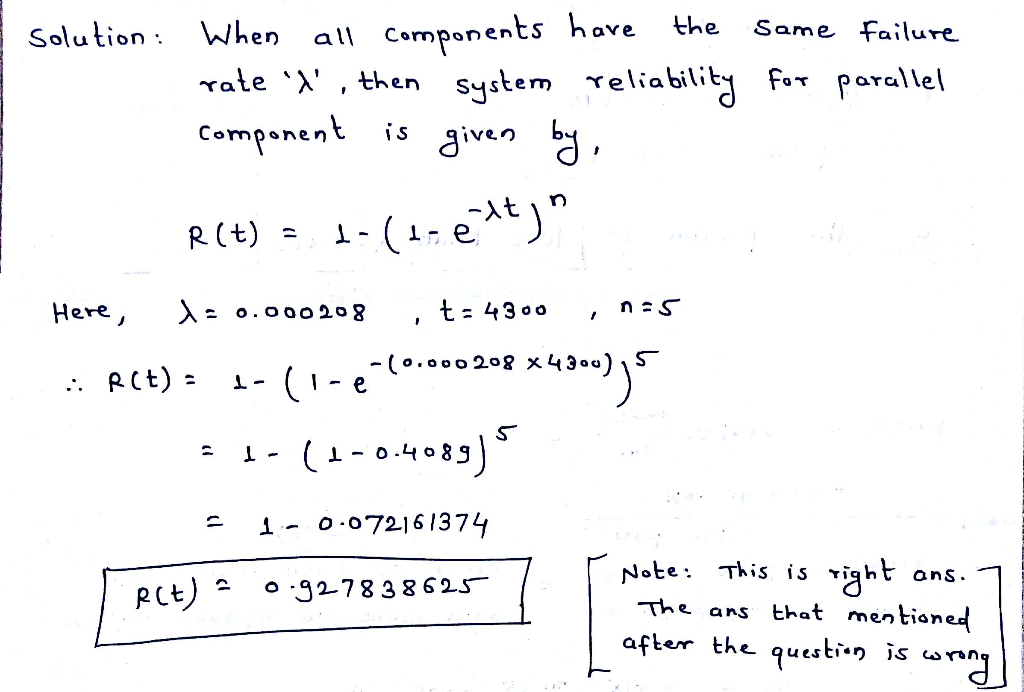Formula is right. You can calculate the value using formula and check the answer.

#### Earn Coins

Coins can be redeemed for fabulous gifts.

Similar Homework Help Questions
• ### Compute the reliability of a system with 7 components, neading with at least 2 in full...

Compute the reliability of a system with 7 components, neading with at least 2 in full operation with the MTTF of each component being 2100 hours, after 2510 hours of operation Correct Answer: 0.676128323

• ### 5. Consider a three-component standby system in which two units are normally on-line. Both on-lin...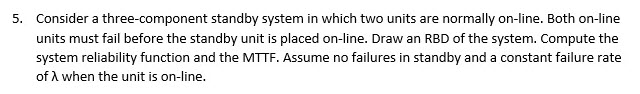5. Consider a three-component standby system in which two units are normally on-line. Both on-line units must fail before the standby unit is placed on-line. Draw an RBD of the system. Compute the system reliability function and the MTTF. Assume no failures in standby and a constant failure rate of A when the unit is on-line. 5. Consider a three-component standby system in which two units are normally on-line. Both on-line units must fail before the standby unit is placed...

• ### Five identical units are arranged in a standby redundant configuration to form a subsystem. Unit failure...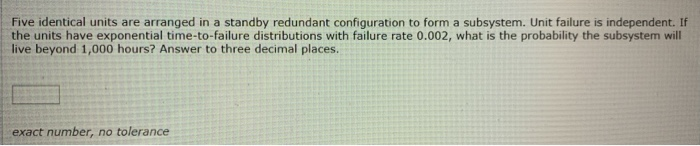Five identical units are arranged in a standby redundant configuration to form a subsystem. Unit failure is independent. If the units have exponential time-to-failure distributions with failure rate 0.002, what is the probability the subsystem will live beyond 1,000 hours? Answer to three decimal places. exact number, no tolerance

• ### 4. Reliability of Systems - Take n components to have failure times Ti, T2, ..., Tn...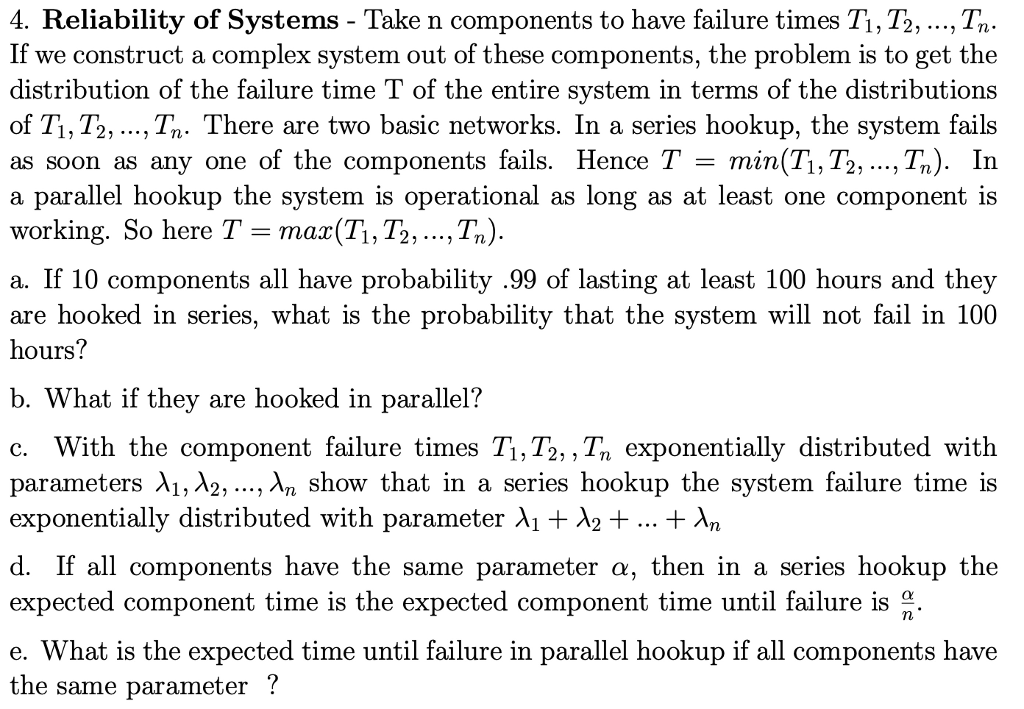4. Reliability of Systems - Take n components to have failure times Ti, T2, ..., Tn If we construct a complex system out of these distribution of the failure time T of the entire svstem in terms of the distributions of Ti, T2, ..., Tn. There are two basic networks. In a series hookup, the system fails as soon as any one of the components fails. Hence T - min(T1, T2, ...,Tn). In a parallel hookup the system is operational...

• ### A system is made up of four independent components in series each having a failure rate...

A system is made up of four independent components in series each having a failure rate of .005 failures per hour. If time to failure is exponential, then the reliability of the system at 10 hours is? (round to 4 decimal places)

• ### 3) Calculate the reliability of the system in Fig. 3 for a 100 hrs. mission. Identical...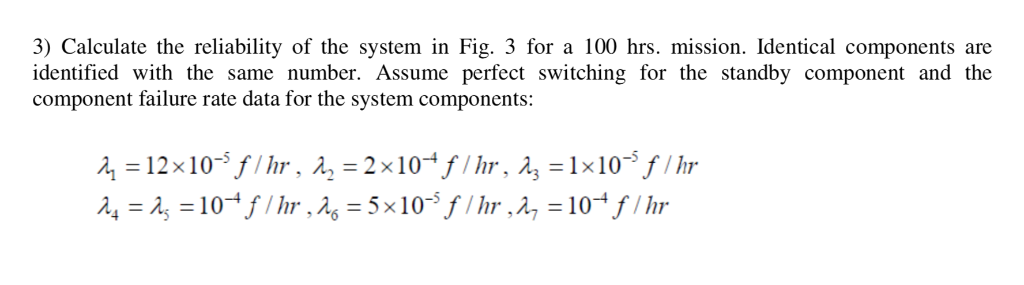3) Calculate the reliability of the system in Fig. 3 for a 100 hrs. mission. Identical components are identified with the same number. Assume perfect switching for the standby component and the component failure rate data for the system components 2/3 Figure 3

• ### A standby mechanical system is composed of three independent and identical motors (i.e., one unit operating...

A standby mechanical system is composed of three independent and identical motors (i.e., one unit operating and the other two on standby). The motor constant failure rate is 0.0009 failures/hour. Calculate the system reliability for a 200-hour mission for this mechanical system.

• ### Question 3 20 pts A system has three components with reliability values A, B, and C....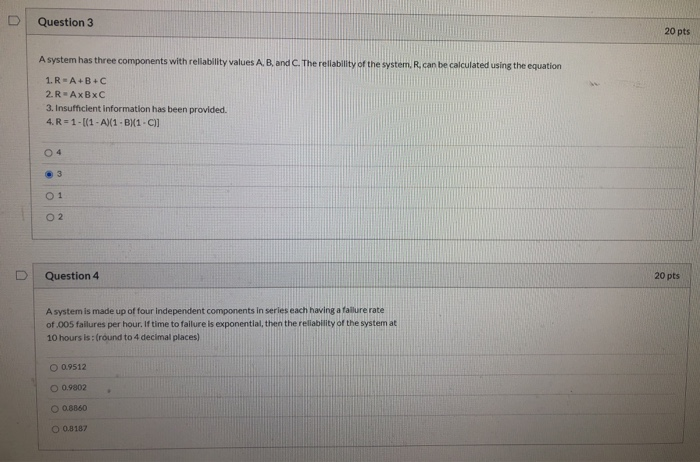Question 3 20 pts A system has three components with reliability values A, B, and C. The reliability of the system. R. can be calculated using the equation 1.R - A+B+C 2.R-AxBxC 3. Insufficient Information has been provided. 4. R = 1 - (1 - A)(1-B1-C)] 04 O 2 U Question 4 20 pts A system is made up of four independent components in series each having a failure rate of .005 failures per hour. If time to failure is...

• ### A system composes of three components. These components have constant failure rates of 0.0004, 0....

A system composes of three components. These components have constant failure rates of 0.0004, 0.0005, 0.0003 failures per hour. The system will stop working, if any one of its components fails. Calculate the following: 1. The reliability of the system at 2500 hour running time? 2. The system hazard rate? 3. Mean time to failure of the system?

• ### Consider a system typically contain components in both serial and parallel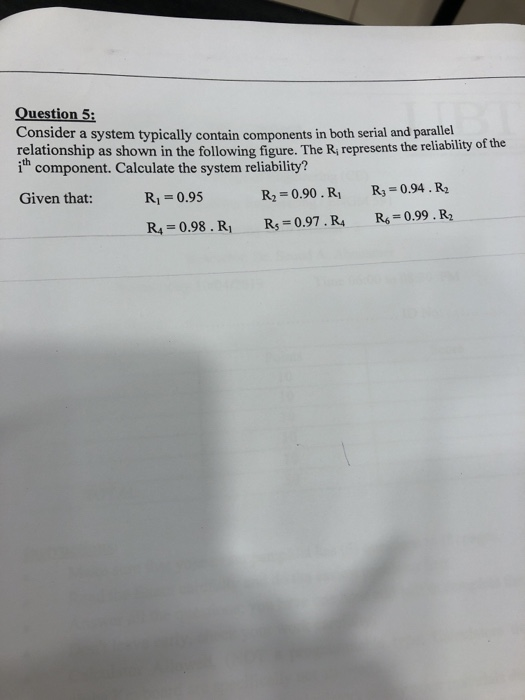consider a system typically contain components in both serial and parallel Question 5: Consider a system typically contain components in both serial and parallel relationship as shown in the following figure. The R, represents the reliability of the ith component. Calculate the system reliability? Given that: R1-0.95 R2-0.90.R R 0.94.R2 Question 5: Consider a system typically contain components in both serial and parallel relationship as shown in the following figure. The R, represents the reliability of the ith component. Calculate...